# Safe Flexible Hypothesis Tests for Practical Scenarios

Safe tests is a collective name for a new form of hypothesis tests that are based on e-values (instead of p-values). The original paper on e-values by Grunwald, de Heide and Koolen can be found here. For each hypothesis testing setting where one would normally use a p-value, a safe test can be designed, with a number of advantages that are elaborately described and illustrated in this vignette. Currently, this package provide safe tests for the z-test, t-test, Fisher’s exact test, the chi-squared test (the safe test of 2 proportions), and the logrank test for survival data. In this vignette, we will illustrate the concepts of safe testing and e-values through the t-test as an example. This safe test is designed to be GROW; It is designed to, on average, detect the effect as quickly as possible, if the effect actually exists.

Technically, E-variables, are non-negative random variables (test statistics) that have an expected value of at most one under the null hypothesis. The E-variable can be interpreted as an gamble against the null hypothesis in which an investment of 1$returns E$ whenever the null hypothesis fails to hold true. Hence, the larger the observed e-value, the larger the incentive to reject the null (see the original paper).

A big advantage of e-values over their p-value equivalents is that safe tests conserve the type I error guarantee (false positive rate) regardless of the sample size. This implies that the evidence can be monitored as the observations come in, and the researcher is allowed to stop the experiment early (optional stopping) without over-inflating the chance of a false discovery. By stopping early fewer participants will be put at risk. In particular, those patients who are assigned to the control condition, when a treatment is effective. Safe tests also allow for optional continuation, that is the extension of an experiment regardless of the motivation. For instance, if more funds become available, or if the evidence looks promising and the funding agency, a reviewer, or an editor urges the experimenter to collect more data.

Importantly, for the safe tests presented here neither optional stopping nor continuation leads to the test exceeding the tolerable type I error $$\alpha$$. As the results do not depend on the planned, current, or future sample sizes, safe tests allow for anytime valid inferences. We illustrate these properties below.

Firstly, we show how to design an experiment based on safe tests for testing means.

Secondly, simulations are run to show that safe tests indeed conserve the type I error guarantee under optional stopping for testing means. We also show that optional stopping causes the false null rejection rate of the classical p-value test to exceed the tolerable level $$\alpha$$ type I error guarantee. In other words, with classical tests one cannot adapt to the information acquired during the study without increasing the risk of making a false discovery.

Lastly, it is shown that optionally continuing non-significant experiments also causes the p-value tests to exceed the promised level $$\alpha$$ type I error guarantee, whereas this is not the case for safe tests.

This demonstration further emphasises the rigidity of experimental designs when inference is based on a classical test: the experiment cannot be stopped early, nor extended. Thus, the planned sample size has to be final. As such, a rigorous protocol needs to account for possible future sample sizes, which is practically impossible. Even if such a protocol can be made, there is no guarantee that the experiments go exactly according to plan, as things might go wrong during the study.

The ability to act on information that accumulates during the study –without sacrificing the correctness of the resulting inference– was the main motivation for the development of safe tests, as it provides experimenters with the much needed flexibility.

## Installation

The stable version can be installed by entering in R:

install.packages("safestats")

The development version can be found on GitHub, which can be installed with the remotes package from CRAN by entering in R:

remotes::install_github("AlexanderLyNL/safestats", build_vignettes = TRUE)

The command

library(safestats)

# 1. Designing safe t-test experiments

## Type I error and type II errors

To avoid bringing an ineffective medicine to the market, experiments need to be conducted in which the null hypothesis of no effect is tested. Here we show how flexible experiments based on safe tests can be designed.

As the problem is statistical in nature, due to variability between patients, we cannot guarantee that all of the medicine that pass the test will indeed be effective. Instead, the target is to bound the type I error rate by a tolerable $$\alpha$$, typically, $$\alpha = 0.05$$. In other words, at most 5 out of the 100 ineffective drugs are allowed to pass the safe test.

At the same time, we would like to avoid a type II error, that is, missing out on finding an effect, when there is one. Typically, the targetted type II error rate is $$\beta = 0.20$$, which implies that whenever there truly is an effect, an experiment needs to be designed in such a way that the effect is detect with $$1 - \beta =$$ 80% chance.

## Case (I): Designing experiments with the minimal clinically relevant effect size known

Not all effects are equally important, especially, when a minimal clinically relevant effect size can be formulated. For instance, suppose that a population of interest has a population average systolic blood pressure of $$\mu = 120$$ mmHg (milimetre of mercury) and that the population standard deviation is $$\sigma = 15$$. Suppose further that all approved blood pressure drugs change the blood pressure by at least 9 mmHg, then a minimal clinically relevant effect size can be specified as $$\delta_{\min} = (\mu_{\text{post}} - \mu_{\text{pre}}) / (\sqrt{2} \sigma) = 9 / (15 \sqrt{2} ) = 0.42$$, where $$\mu_{\text{post}}$$ represents the average blood pressure after treatment and $$\mu_{\text{pre}}$$ the average blood pressure before treatment of the population of interest. The $$\sqrt{2}$$-term in the denominator is a result of the measurements being paired.

Based on a tolerable type I error rate of $$\alpha = 0.05$$, type II error rate of $$\beta = 0.20$$, and minimal clinical effect size of $$\delta_{\min} \approx 0.42$$, the function designSafeT allows us to design the experiment as follows.

alpha <- 0.05
beta <- 0.2
deltaMin <- 9/(sqrt(2)*15)
designObj <- designSafeT(deltaMin=deltaMin, alpha=alpha, beta=beta,
alternative="greater", testType="paired", seed=1, pb=FALSE)
designObj
#>
#>  Safe Paired Sample T-Test Design
#>
#>               n1Plan±2se, n2Plan±2se = 54±3.69867, 54±3.69867
#> minimal standardised mean difference = 0.4242641
#>                          alternative = greater
#>                      power: 1 - beta = 0.8
#>                    parameter: deltaS = 0.4242641
#>                                alpha = 0.05
#>     decision rule: e-value > 1/alpha = 20
#>
#> Timestamp: 2022-01-20 21:24:12 CET
#>
#> Note: If it is only possible to look at the data once, then n1Plan = 68 and n2Plan = 68.

The design object defines both the parameter deltaS that will used to compute the e-value, e.g., 0.4242641, and the planned sample size(s), e.g., 54, 54. Hence, in this case we need the pre- and post-measurements of about 54 patients to detect a true effect of $$\delta=\delta_{\min} \approx 0.42$$. This nPlan of 54 is based on continuously monitoring the e-value and stopping the experiment as soon as it exceeds $$1/\alpha = 20$$. Note that the event that the E-variable exceeds $$1/\alpha$$ is random, and the sample size at which this occurs is therefore also random. This randomness is expressed with nPlan being reported with two standard error of the mean. When it is only possible to conduct the test once, when the data are treated as a single batch, then 68 patients (thus 14 more) are needed to detect $$\delta=\delta_{\min} \approx 0.42$$ with 80% chance.

## Case (II): Minimal clinically relevant effect size unknown, but maximum number of samples known.

It is not always clear what the minimal clinically relevant effect size is. Suppose that the tolerable type I and type II error rates and a maximum sample size nMax, say, 100 is known due to budget constraints. In this case, the design function can be called with a reasonable range of minimal clinically relevant effect sizes, and a prospective futility analysis can be done:

# Recall:
# alpha <- 0.05
# beta <- 0.2
plotSafeTSampleSizeProfile <- plotSafeTDesignSampleSizeProfile(alpha=alpha, beta=beta,
lowDeltaMin=0.1, highDeltaMin=1,
nMax=100, seed=1, alternative="greater",
testType="paired", nSim=1000, pb=FALSE)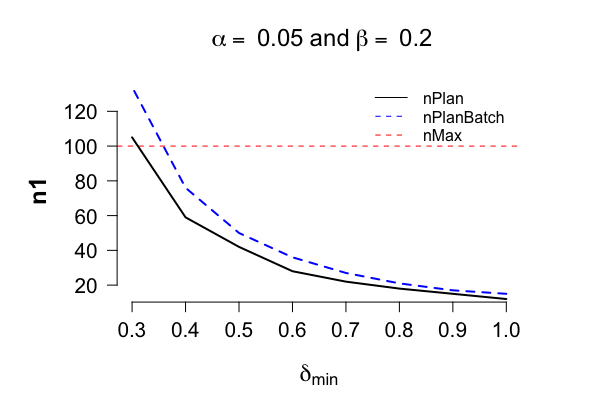plotSafeTSampleSizeProfile$deltaDomain #>  1.0 0.9 0.8 0.7 0.6 0.5 0.4 0.3 plotSafeTSampleSizeProfile$allN1PlanSafe
#>   12  15  18  22  28  42  59 105

The plot shows that when we have budget for at most 100 paired samples, we can only guarantee a power of 80%, if the true effect size is at least 0.35. If a field expert believes that an effect size of 0.3 is realistic, then the plot shows that we should either apply for extra grant money to test an additional 5 patients, or decide that it’s futile to conduct this experiment, and that we should spend our time and efforts on different endeavours instead.

# 2. Inference with safe tests: Optional stopping

In this section we illustrate the operational characteristics of the safe t-test under optional stopping. The next section studies the operational characteristics of this test under optional continuation. Robustness to these both optional stopping and continuation demonstrate that if the null hypothesis of no effect holds true, then there is less than $$\alpha$$ chance that the E-variable will ever reject the null. After illustrating the operational characteristics of the safe test under the null, we then demonstrate its performance under the alternative.

## Safe tests conserve the type I error rate: Batch analysis

We first show that the type I error is preserved for the batch analysis, that is, when the data are only analysed once at nPlan.

set.seed(1)
preData <- rnorm(n=designObj$nPlan, mean=120, sd=15) postData <- rnorm(n=designObj$nPlan, mean=120, sd=15)
# Thus, the true delta is 0:
# deltaTrue <- (120-120)/(sqrt(2)*15)
safeTTest(x=preData, y=postData, alternative = "greater",
designObj=designObj, paired=TRUE)
#>
#>  Safe Paired Sample T-Test
#>
#> data:  preData and postData. n1 = 54, n2 = 54
#> estimates: mean of the differences = -1.1824
#> 95 percent confidence sequence:
#>  -9.952138  7.587318
#>
#> test: t = -0.42559, deltaS = 0.42426
#> e-value = 0.0020649 > 1/alpha = 20 : FALSE
#> alternative hypothesis: true difference in means ('x' minus 'y') is greater than 0
#>
#> design: the test was designed with alpha = 0.05
#> for experiments with n1Plan = 54, n2Plan = 54
#> to guarantee a power = 0.8 (beta = 0.2)
#> for minimal relevant standardised mean difference = 0.42426 (greater)

or equivalently with syntax closely resembling the standard t.test code in R:

safe.t.test(x=preData, y=postData, alternative = "greater",
designObj=designObj, paired=TRUE)
#>
#>  Safe Paired Sample T-Test
#>
#> data:  preData and postData. n1 = 54, n2 = 54
#> estimates: mean of the differences = -1.1824
#> 95 percent confidence sequence:
#>  -9.952138  7.587318
#>
#> test: t = -0.42559, deltaS = 0.42426
#> e-value = 0.0020649 > 1/alpha = 20 : FALSE
#> alternative hypothesis: true difference in means ('x' minus 'y') is greater than 0
#>
#> design: the test was designed with alpha = 0.05
#> for experiments with n1Plan = 54, n2Plan = 54
#> to guarantee a power = 0.8 (beta = 0.2)
#> for minimal relevant standardised mean difference = 0.42426 (greater)

The following code replicates this simulation a 1,000 times and shows that in only a few cases will the E-variable cross the boundary of $$1/\alpha$$ under the null:

# alpha <- 0.05

set.seed(1)
eValues <- replicate(n=1000, expr={
preData <- rnorm(n=designObj$nPlan, mean=120, sd=15) postData <- rnorm(n=designObj$nPlan, mean=120, sd=15)
safeTTest(x=preData, y=postData, alternative = "greater",
designObj=designObj, paired=TRUE)$eValue} ) mean(eValues > 20) #>  0.009 mean(eValues > 20) < alpha #>  TRUE Thus, in this simulation with the null hypothesis holding true and if the safe test is only conducted once at the planned sample size, then in 9 out of 1,000 experiments the null hypothesis was falsely rejected. ## Safe tests allow for early stopping without inflating the type I error rate above the tolerable $$\alpha$$-level What makes the safe tests in this package particularly interesting is that they allow for early stopping without the test exceeding the tolerable type I error rate of $$\alpha$$. This means that the e-value can be monitored as the data come in, and when there is a sufficient amount of evidence against the null, i.e., whenever e-value$ > 1/$, the experiment can be stopped early. This puts fewer patients at risk, and allows for more efficient scientific scrutiny. Note that not all E-variables necessarily allow for optional stopping: this only holds for some special E-variables, that are also test martingales. More information can be found, for example, in the first author’s master thesis, Chapter 5. ### Optional stopping does not causes safe tests to over-reject the null, but is problematic for p-values Optionally stopping results in the type I error rate of the safe test to not exceed the tolerable $$\alpha$$ level, whereas tracking the classical p-value tests and acting on it does result in an overinflation of the type I error. In other words, optional stopping with these p-value tests leads to an increased risk of falsely claiming that a medicine is effective, while in reality it is not. The following code replicates 500 experiments and each data set is generated with a true effect size set to zero. For each data set a sequential analysis is run, that is, an e-value is computed as the data come in. As soon as the e-value exceeds $$1 / \alpha = 20$$, the null is rejected and the experiment can be stopped. # Recall: # alpha <- 0.05 # beta <- 0.2 freqDesignObj <- designFreqT(deltaMin=deltaMin, alpha=alpha, beta=beta, alternative="greater", testType="paired") nSim <- 500 simResultDeltaTrueIsZero <- simulate(object=designObj, nSim=nSim, seed=1, deltaTrue=0, freqOptioStop=TRUE, nPlanFreq=freqDesignObj$nPlan,
muGlobal=120, sigmaTrue=15, pb=FALSE)
simResultDeltaTrueIsZero
#>
#>    Simulations for Safe Paired Sample T-Test
#>
#> Based on nSim = and if the true effect size is
#>     deltaTrue = 0
#> then the safe test optimised to detect an effect size of at least:
#>     deltaMin = 0.4242641
#> with tolerable type I error rate of
#>     alpha = 0.05 and power: 1-beta = 0.8
#> For experiments with planned sample sizes:
#>     n1Plan = 54 and n2Plan = 54
#>
#> Is estimated to have a null rejection rate of
#>     powerAtNPlan = 0.002
#> at the planned sample sizes.
#> For the p-value test:    freqPowerAtNPlan = 0.05
#>
#> Is estimated to have a null rejection rate of
#>     powerOptioStop = 0.026
#> under optional stopping, and the average stopping time is:
#>     n1Mean = 53.268
#> For the p-value test:    freqPowerOptioStop = 0.21

Note that optional stopping always increases the chance of observing a false detection. For the safe test this increased to 2.6%, which is still below the tolerable 5%. On the other hand, tracking the p-value and rejecting the null as soon it falls below $$\alpha$$ leads to 21%, which is well above 5%.

## Safe tests detect the effect early if it is present: deltaTrue equal to deltaMin

In this section we illustrate the operational characteristics of the safe t-test under optional stopping, when the effect is present. The following code replicates 500 experiments and each data set is generated with a true effect size that equals the minimal clinical-relevant effect size of $$\delta_{\min}=9/(15 \sqrt{2}) \approx 0.42$$. If the e-value does not exceed $$1 / \alpha$$, the experiment is run until all samples are collected as planned.

# Recall:
# alpha <- 0.05
# beta <- 0.2
deltaMin <- 9/(sqrt(2)*15)      # = 0.42
simResultDeltaTrueIsDeltaMin <- simulate(object=designObj, nSim=nSim,
seed=1, deltaTrue=deltaMin,
muGlobal=120, sigmaTrue=15, pb=FALSE)
simResultDeltaTrueIsDeltaMin
#>
#>    Simulations for Safe Paired Sample T-Test
#>
#> Based on nSim = and if the true effect size is
#>     deltaTrue = 0.4242641
#> then the safe test optimised to detect an effect size of at least:
#>     deltaMin = 0.4242641
#> with tolerable type I error rate of
#>     alpha = 0.05 and power: 1-beta = 0.8
#> For experiments with planned sample sizes:
#>     n1Plan = 54 and n2Plan = 54
#>
#> Is estimated to have a null rejection rate of
#>     powerAtNPlan = 0.722
#> at the planned sample sizes.
#>
#> Is estimated to have a null rejection rate of
#>     powerOptioStop = 0.808
#> under optional stopping, and the average stopping time is:
#>     n1Mean = 33.41

The simulations confirms that at the planned sample size there is indeed about 80% chance of detecting the minimal clinically relevant effect. The discrepancy is due to sampling error and vanishes as the number of simulations increases.

To see the distributions of stopping times, the following code can be run

plot(simResultDeltaTrueIsDeltaMin)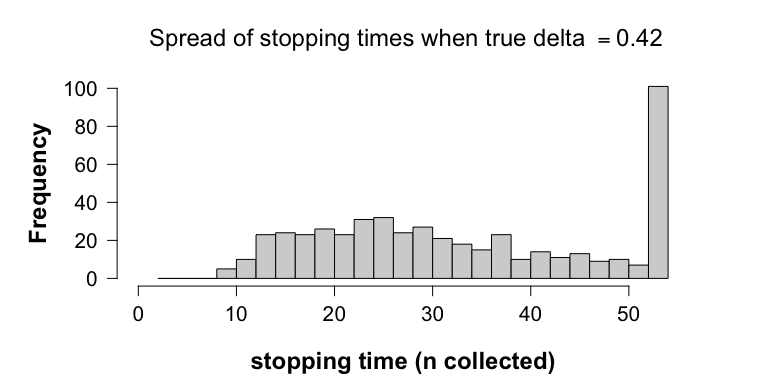tabResult <- table(simResultDeltaTrueIsDeltaMin$safeSim$allN)
tabResult
#>
#>  10  11  12  13  14  15  16  17  18  19  20  21  22  23  24  25  26  27  28  29
#>   5   4   6   6  17  13  11   8  15  14  12  11  12  18  13  14  18  13  11  14
#>  30  31  32  33  34  35  36  37  38  39  40  41  42  43  44  45  46  47  48  49
#>  13  10  11   7  11   4  11  12  11   4   6  10   4   6   5   4   9   6   3   5
#>  50  51  52  54
#>   5   4   3 101

The table shows the full distribution of the times at which the experiment is stopped. For instance, 210 out of the 500 experiments stopped before half the planned sample size. In these cases we were lucky and the effect was detected early. The last bar collects all experiments that ran until the planned sample sizes, thus, also those that did not lead to a null rejection at n=54. To see the distributions of stopping times of only the experiments where the null is rejected, we run the following code:

plot(simResultDeltaTrueIsDeltaMin, showOnlyNRejected=TRUE)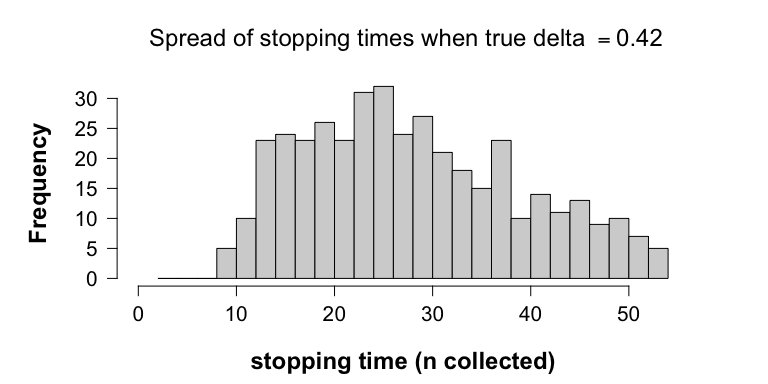## Safe tests detect the effect even earlier if it is larger than expected

What we believe is clinically minimally relevant might not match reality. One advantage of safe tests is that they perform even better, if the true effect size is larger than the minimal clinical effect size that is used to plan the experiment. This is illustrated with the following code

# Recall:
# alpha <- 0.05
# beta <- 0.2
# deltaMin <- 9/(sqrt(2)*15)      # = 0.42
deltaTrueLarger <- 0.6
simResultDeltaTrueLargerThanDeltaMin <- simulate(object=designObj,
nSim=nSim, seed=1,
deltaTrue=deltaTrueLarger,
muGlobal=120, sigmaTrue=15, pb=FALSE)
simResultDeltaTrueLargerThanDeltaMin
#>
#>    Simulations for Safe Paired Sample T-Test
#>
#> Based on nSim = and if the true effect size is
#>     deltaTrue = 0.6
#> then the safe test optimised to detect an effect size of at least:
#>     deltaMin = 0.4242641
#> with tolerable type I error rate of
#>     alpha = 0.05 and power: 1-beta = 0.8
#> For experiments with planned sample sizes:
#>     n1Plan = 54 and n2Plan = 54
#>
#> Is estimated to have a null rejection rate of
#>     powerAtNPlan = 0.974
#> at the planned sample sizes.
#>
#> Is estimated to have a null rejection rate of
#>     powerOptioStop = 0.986
#> under optional stopping, and the average stopping time is:
#>     n1Mean = 22.646

With a larger true effect size, the power increased to 98.6%. More importantly, this increase is picked up earlier by the designed safe test, and optional stopping allows us to act on this. Note that the average stopping time is now decreased, from 33.41 to 22.646. This is apparent from the fact that the histogram of stopping times is now shifted to the left:

plot(simResultDeltaTrueLargerThanDeltaMin)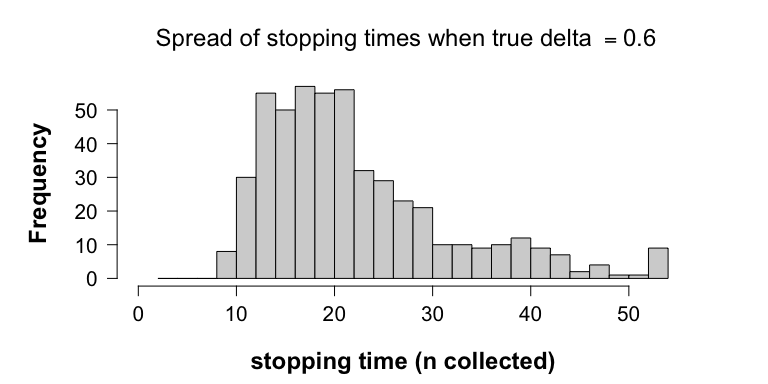Hence, this means that if the true effect is larger than what was planned for, the safe test will detect this larger effect earlier on, which results in a further increase of efficiency.

The last scenario with deltaTrue smaller than what was planned for, that is, deltaMin, is discussed in the context of optional continuation.

# 3. Optional Continuation

## Optional continuation is problematic for p-values and leads to overinflating the type I error rate

Firstly, we show that optional continuation also causes p-values to over-reject the null. In the following we consider the situation in which we continue studies for which a first batch of data resulted in $$p \geq \alpha$$. These non-significant experiments are extended with a second batch of data with the same sample sizes as the first batch, that is, 36, 36. We see that selectively continuing non-significant experiments causes the collective rate of false null rejections to be larger than $$\alpha$$.

The following code simulates 500 (first batch) experiments under the null, each with the same (frequentist) sample sizes as planned for, resulting in 500 p-values:

dataBatch1 <- generateNormalData(nPlan=freqDesignObj$nPlan, deltaTrue=0, nSim=nSim, paired=TRUE, seed=1, muGlobal=120, sigmaTrue=15) pValuesBatch1 <- vector("numeric", length=nSim) for (i in seq_along(pValuesBatch1)) { pValuesBatch1[i] <- t.test(x=dataBatch1$dataGroup1[i, ],
y=dataBatch1$dataGroup2[i, ], alternative="greater", paired=TRUE)$p.value
}
mean(pValuesBatch1 > alpha)
#>  0.968
sum(pValuesBatch1 < alpha)
#>  16

Hence, after a first batch of data, we get 16 incorrect null rejections out of a 500 experiments (3.2%).

The following code continues only the non-significant 484 experiments with a second batch of data all also generated under the null, and plots two histograms.

selectivelyContinueDeltaTrueIsZeroWithP <-
selectivelyContinueTTestCombineData(oldValues=pValuesBatch1,
valuesType="pValues",
alternative="greater",
oldData=dataBatch1,
deltaTrue=0,
n1Extra=freqDesignObj$nPlan, n2Extra=freqDesignObj$nPlan,
alpha=alpha,
seed=2, paired=TRUE,
muGlobal=120, sigmaTrue=15)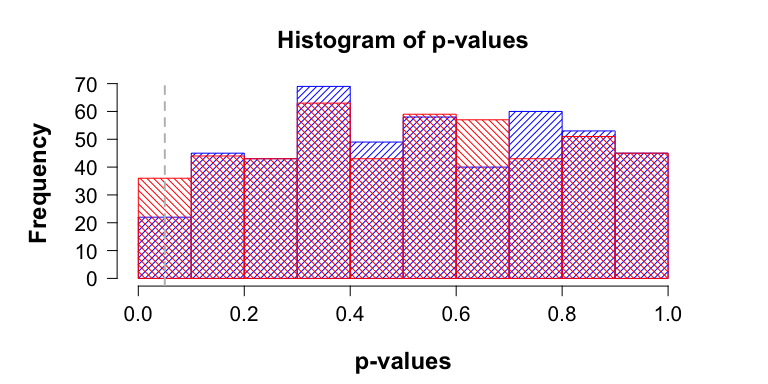The blue histogram represents the distribution of the 484 non-significant p-values calculated over the first batch of data, whereas the red histogram represents the distribution of p-values calculated over the two batches of data combined.

The commands

pValuesBatch1To2 <- selectivelyContinueDeltaTrueIsZeroWithP$newValues sum(pValuesBatch1To2 < alpha) #>  14 show that by extending the non-significant results of the first batch with a second batch of data, we got another 14 false null rejections. This brings the total number of incorrect null rejections to 30 out of 500 experiments, hence, 6%, which is above the tolerable $$\alpha$$-level of 5%. The reason why p-values over-reject the null under optional stopping and optional continuation is due to p-values being uniformly distributed under the null. As such, if the null holds true and the number of samples increases, then the p-value meanders between 0 and 1, thus, eventually crossing any fixed $$\alpha$$-level. ## Two ways to optionally continue studies with safe tests Safe tests, as we will show below, do conserve the type I error rate under optional continuation. Optional continuation implies gathering more samples than was planned for because, for instance, (1) more funding came available and the experimenter wants to learn more, (2) the evidence looked promising, (3) a reviewer or editor urged the experimenter to collect more data, or (4) other researchers attempt to replicate the first finding. A natural way to deal with the first three cases is by computing an e-value over the combined data set. This is permitted if the data come from the same population, and if the E-variable used is a test martingale, which is the case for the problem at hand. Replication attempts, however, are typically based on samples from a different population. One way to deal with this is by multiplying the e-value computed from the original study with the e-value computed from the replication attempt. In this situation, the e-value formula for the replication study could also be redesigned through the function, for example when more information on nuisance parameters or effect size has become available for designing a more powerful test. We show that both procedures are safe, that is, they do not lead to exceedance of the tolerable type I error rate, as was the case with classical p-values. ### i. Optional continuation by extending the experiment does not result in safe tests exceeding the tolerable $$\alpha$$-level In this subsection, we show that only continuing studies for which the e-value $$\leq 1/ \alpha$$ does not lead to an over-rejection of the null. This is because the sampling distribution of e-values under the null slowly drifts towards smaller values as the number of samples increases. Again, we consider the situation in which we only continue studies for which the original e-values did not lead to a null rejection. For the first batch of e-values, we use the simulation study ran in the previous section, and we recall that under optional stopping we get dataBatch1 <- list(dataGroup1=simResultDeltaTrueIsZero$safeSim$dataGroup1, dataGroup2=simResultDeltaTrueIsZero$safeSim$dataGroup2) eValuesBatch1 <- simResultDeltaTrueIsZero$safeSim$eValues sum(eValuesBatch1 > 1/alpha) #>  13 thus, 13 false null rejections out of 500 experiments. The follow-up batches of data will be of the same size as the original, thus, 54, 54, and will also be generated under the null. The slow drift to lower e-values is visualised by two histograms. The blue histogram represents the sampling distribution of e-values of the original simulation study that did not resulted in a null rejection. The red histogram represents the sampling distribution of e-values computed over the two batches of data combined. To ease visualisation, we plot the histogram of the logarithm of e-values; a negative log e-value implies that the e-value is smaller than one, whereas a positive log e-values corresponds to e-values larger than one. For this we run the following code: selectivelyContinueDeltaTrueIsZero <- selectivelyContinueTTestCombineData(oldValues=eValuesBatch1, designObj=designObj, alternative="greater", oldData=dataBatch1, deltaTrue=0, seed=2, paired=TRUE, muGlobal=120, sigmaTrue=15, moreMainText="Batch 1-2")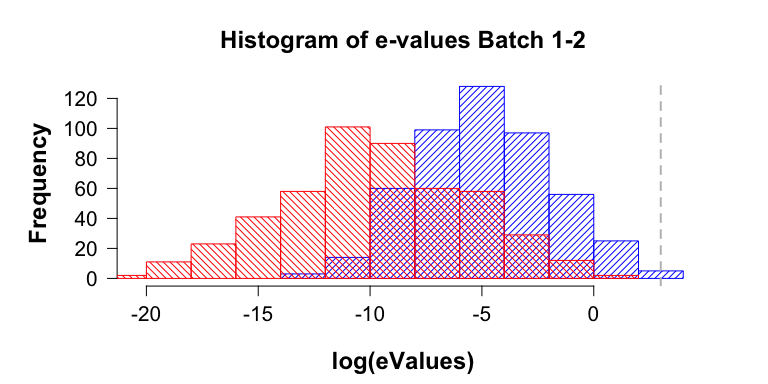Note that compared to blue histogram, the red histogram is shifted to the left, thus, the sampling distribution of e-values computed over the two batches combined concentrates on smaller values. In particular, most of the mass remains under the threshold value of $$1/\alpha$$, which is represented by the vertical grey line $$\log(1/\alpha) \approx 3.00$$. This shift to the left is caused by the increase in sample sizes from n1=n2=54 to n1=n2=108. The commands eValuesBatch1To2 <- selectivelyContinueDeltaTrueIsZero$newValues
sum(eValuesBatch1To2 > 1/alpha)
#>  0
length(eValuesBatch1To2)
#>  487

show that 0 out of the 487 of the selectively continued experiments (0%) now lead to a false null rejection due to optional continuation. Hence, after the second batch of data the total number of total number of false null rejections is 13 out of a total of a 500 original experiments, thus, 2.6%. Similar, a third batch won’t increase the collective false rejection rate either.

eValuesBatch1To2 <- selectivelyContinueDeltaTrueIsZero$newValue dataBatch1To2 <- selectivelyContinueDeltaTrueIsZero$combinedData

selectivelyContinueDeltaTrueIsZero <-
selectivelyContinueTTestCombineData(oldValues=eValuesBatch1To2,
designObj=designObj,
alternative="greater",
oldData=dataBatch1To2,
deltaTrue=0,
seed=3, paired=TRUE,
muGlobal=120, sigmaTrue=15,
moreMainText=paste("Batch: 1 to", 3))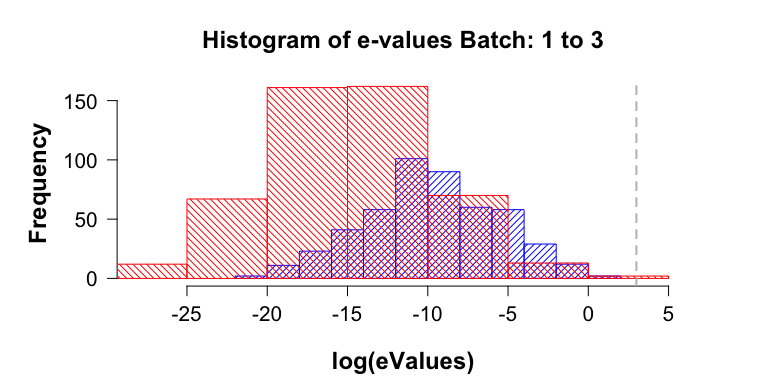sum(selectivelyContinueDeltaTrueIsZero$newValues > 1/alpha) #>  1 Another batch yields e-values so small that it leads to an underflow. #### When the effect is present optional continuation results in safe tests correctly rejecting the null The slow drift of the sampling distribution of e-values to smaller values is replaced by a fast drift to large values whenever there is an effect. We again consider the situation in which we continue studies for which the first batch of e-values did not lead to a null rejection. For this we consider the case with deltaTrue smaller than deltaMin. simResultDeltaTrueLessThanDeltaMin <- simulate(object=designObj, nSim=1000L, seed=1, deltaTrue=0.3, muGlobal=120, sigmaTrue=15, pb=FALSE) simResultDeltaTrueLessThanDeltaMin #> #> Simulations for Safe Paired Sample T-Test #> #> Based on nSim = and if the true effect size is #> deltaTrue = 0.3 #> then the safe test optimised to detect an effect size of at least: #> deltaMin = 0.4242641 #> with tolerable type I error rate of #> alpha = 0.05 and power: 1-beta = 0.8 #> For experiments with planned sample sizes: #> n1Plan = 54 and n2Plan = 54 #> #> Is estimated to have a null rejection rate of #> powerAtNPlan = 0.354 #> at the planned sample sizes. #> #> Is estimated to have a null rejection rate of #> powerOptioStop = 0.502 #> under optional stopping, and the average stopping time is: #> n1Mean = 43.126 The follow-up batch of data will again be of the same sizes, thus, 54, 54, and be generated with the same deltaTrue smaller than deltaMin, as in the first batch. As a first batch of e-values, we use the simulation study ran in the previous section when deltaTrue equals deltaMin, and we recall that under optional stopping we get dataBatch1 <- list( dataGroup1=simResultDeltaTrueLessThanDeltaMin$safeSim$dataGroup1, dataGroup2=simResultDeltaTrueLessThanDeltaMin$safeSim$dataGroup2 ) eValuesBatch1 <- simResultDeltaTrueLessThanDeltaMin$safeSim$eValues sum(eValuesBatch1 > 1/alpha) #>  251 251 correct null rejections, since this simulation is based on data generated under alternative with deltaTrue > 0. The following code selectively continues the 249 experiments which did not lead to a null rejection: selectivelyContinueDeltaTrueLessThanDeltaMin <- selectivelyContinueTTestCombineData(oldValues=eValuesBatch1, designObj=designObj, alternative="greater", oldData=dataBatch1, deltaTrue=deltaMin, seed=2, paired=TRUE, muGlobal=120, sigmaTrue=15)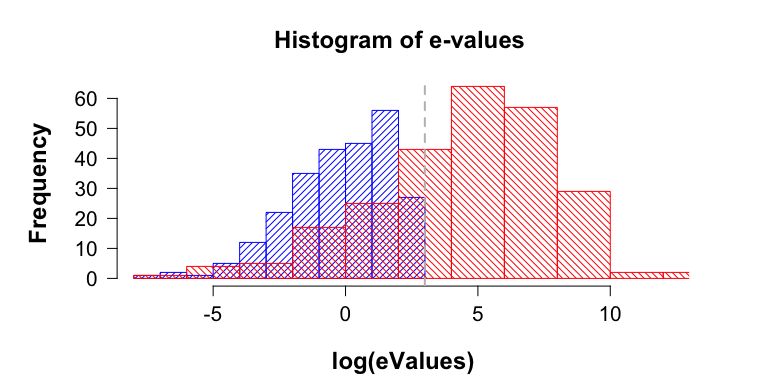The plot shows that after the second batch of data that the sampling distribution of e-values now concentrates on larger values, as is apparent from the blue histogram shifting to the red histogram on the right. Note that most of the red histogram’s mass is on the right-hand side of the grey vertical line that represents the $$\alpha$$ threshold (e.g., $$\log(1/\alpha) \approx 3$$. The continuation of the 249 experiments with $$E < 1/\alpha=20$$ led to eValuesBatch1To2 <- selectivelyContinueDeltaTrueLessThanDeltaMin$newValues
sum(eValuesBatch1To2 > 1/alpha)
#>  177

an additional 177 correct null rejections (71.0843373% of 249 experiments). This brings up the total number of null rejections to 428 out of the 500 experiments. In this case, a null rejection is correct, since the data were generated with a true effect larger than zero, though smaller than the minimal clinically relevant effect size.

### ii. Optional continuation through replication studies

It is not always appropriate to compute one e-value over a combine data set, in particular for replication attempts where the original experiment is performed on a different population. In that case, one can still easily do safe inference by multiplying the e-values computed over each data set separately. This procedure also conserves the $$\alpha$$-level, as we show below.

In all scenarios the simulation results of the optional stopping studies are used as original experiments. The data from these simulated experiments were all generated with a global population mean (e.g., baseline blood pressure) that was set to $$\mu_{g}=120$$, a population standard deviation of $$\sigma=15$$, and a deltaTrue, which depending on the absence or presence of the effect was zero, or equal to deltaMin, respectively.

#### Type I error is guaranteed when multiplying e-values

We continue the e-values from the optional stopping simulation study

eValuesOri <- simResultDeltaTrueIsZero$safeSim$eValues

The code below multiplies these original e-values with e-values observed in a replication attempt with a larger sample size. As in the original study the data are generated under the null. Suppose that for the replication attempt we now administer the same drug to a clinical group that has a lower overall baseline blood pressure of $$\mu_{g}=90$$ mmHg and standard deviation of $$\sigma=6$$.

# Needs to be larger than 1/designObj$n1Plan to have at least two samples # in the replication attempt someConstant <- 1.2 repData <- generateNormalData(nPlan=c(ceiling(someConstant*designObj$nPlan),
ceiling(someConstant*designObj$nPlan)), deltaTrue=0, nSim=nSim, muGlobal=90, sigmaTrue=6, paired=TRUE, seed=2) eValuesRep <- vector("numeric", length=nSim) for (i in seq_along(eValuesRep)) { eValuesRep[i] <- safeTTest(x=repData$dataGroup1[i, ],
y=repData$dataGroup2[i, ], designObj=designObj, alternative="greater", paired=TRUE)$eValue
}
eValuesMultiply <- eValuesOri*eValuesRep
mean(eValuesMultiply > 1/alpha)
#>  0.004

This shows that the type I error (0.4% < $$\alpha=5$$%) is still controlled for, even if the replication attempt is done on a different population. In fact, the $$\alpha$$-level is controlled for regardless of the values of the nuisance parameters (e.g., $$\mu_{g}$$ and $$\sigma$$), or the sample sizes of the replication attempt as long as there are more than 2 data points in any study.

#### Multiplying e-values under the alternative

As original experiments we now take the e-values from the optional stopping simulation study with deltaTrue equal to deltaMin:

eValuesOri <- simResultDeltaTrueIsDeltaMin$safeSim$eValues

The code below multiplies these original e-values with e-values based on replication data, which as in the original study are generated under deltaTrue equal to deltaMin, but with different nuisance parameters, e.g., $$\mu_{g}=110$$ and $$\sigma=50$$, thus, much more spread out than in the original studies. Again the replication attempt is assumed to have a larger sample size.

someConstant <- 1.2

repData <- generateNormalData(nPlan=c(ceiling(someConstant*designObj$nPlan), ceiling(someConstant*designObj$nPlan)),
deltaTrue=deltaMin, nSim=nSim,
muGlobal=110, sigmaTrue=50,
paired=TRUE, seed=2)

eValuesRep <- vector("numeric", length=nSim)

for (i in seq_along(eValuesRep)) {
eValuesRep[i] <- safeTTest(x=repData$dataGroup1[i, ], y=repData$dataGroup2[i, ],
designObj=designObj,
alternative="greater", paired=TRUE)\$eValue
}
eValuesMulti <- eValuesOri*eValuesRep
mean(eValuesMulti > 1/alpha)
#>  0.934

This led to 467 null rejections out of the 500 experiments, which is the correct result as the effect is present in both the original and replication studies.

## Conclusion

We believe that optional continuation is essential for (scientific) learning, as it allows us to revisit uncertain decisions such as ($$p < \alpha$$ and e-value $$> 1/\alpha$$) either by extending an experiment directly, or via replication studies. Hence, we view learning as an ongoing process, which requires that inference becomes more precise as data accumulate. The inability of p-values to conserve the $$\alpha$$-level under optional continuation, however, is at odds with this view –by gathering more data after an initial look, the inference becomes less precise, as the likelihood of the null being true after observing $$p < \alpha$$ increases beyond what is tolerable.

Safe tests on the other hand benefit from more data, as the chance of seeing e-value $$> 1/\alpha$$ (slowly) decreases when the null is true, whereas it (quickly) increases when the alternative is true, as the number of samples increases.